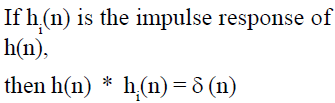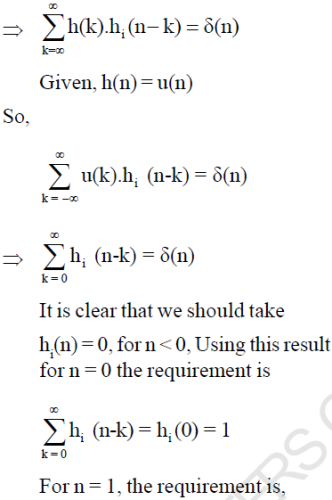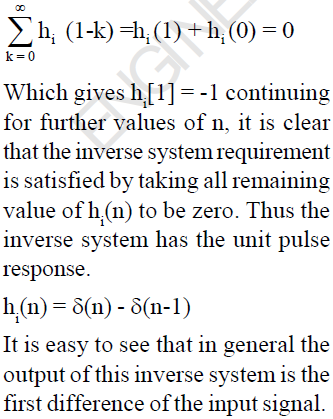Courses

# Signal & Systems

## 25 Questions MCQ Test GATE ECE (Electronics) 2022 Mock Test Series | Signal & Systems

Description
This mock test of Signal & Systems for GATE helps you for every GATE entrance exam. This contains 25 Multiple Choice Questions for GATE Signal & Systems (mcq) to study with solutions a complete question bank. The solved questions answers in this Signal & Systems quiz give you a good mix of easy questions and tough questions. GATE students definitely take this Signal & Systems exercise for a better result in the exam. You can find other Signal & Systems extra questions, long questions & short questions for GATE on EduRev as well by searching above.
QUESTION: 1

### The even part of the signal x(n) = u(n)

Solution:

x(n) = u(n)
x(-n) = u(-n)
Even part of x(n),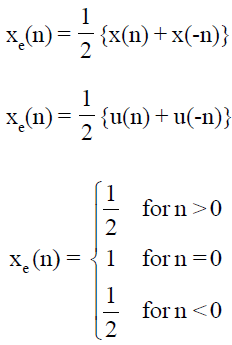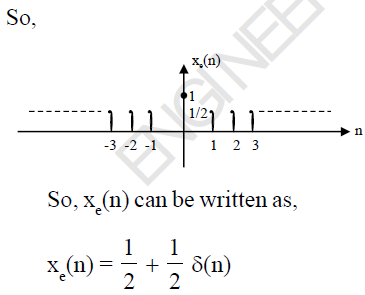QUESTION: 2

### The value of discrete time signal at non-integer is :

Solution:

The value of discrete time signal and non-integers is zero.

QUESTION: 3

### The ROC of the signal x(t) = 4

Solution: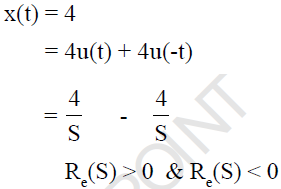Since there is no common ROC is ROC and laplace transform of x(t) does not exist.

QUESTION: 4

The input and output relationship of a system is described as y(t) = ax + b, this system is linear

Solution: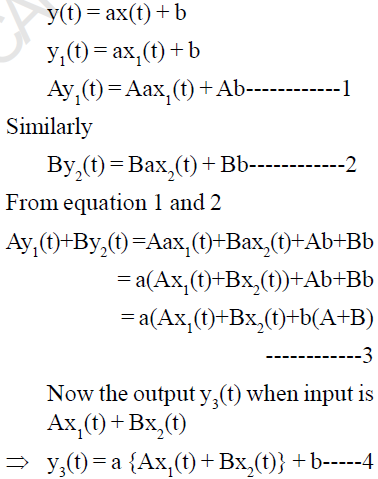For a linear system equation 3 and 4 must be equal, so b = 0, whereas for any value of a.

QUESTION: 5

Which of the following signal is an example of an anti causal signal

Solution:

For an anti causal signal, the value of a signal must be zero for t > 0 so signal is e-at u(-t)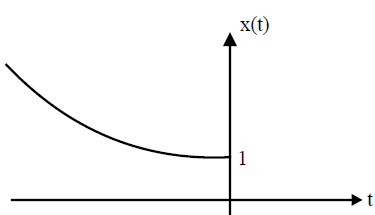QUESTION: 6

The maximum phase-shift provided by the given system is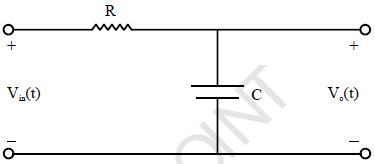Solution: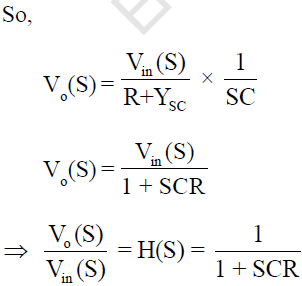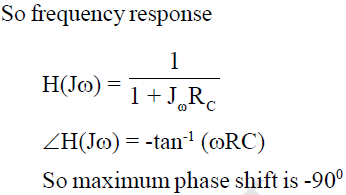QUESTION: 7

Determine the fundamental period of x(n) =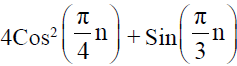Solution: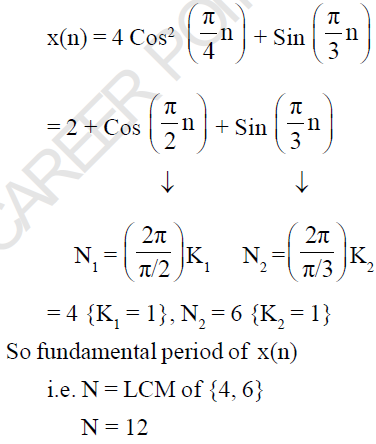QUESTION: 8

Consider a signal x(t) = 4 rect (t/6) and its Fourier - transform is x(ω). Determine the area under the curve in the ω-domain.

Solution: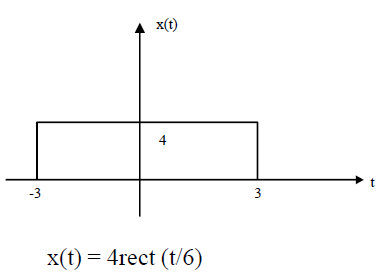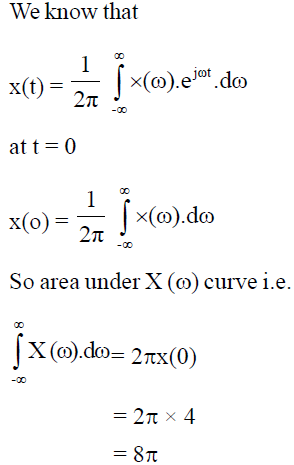QUESTION: 9

If the Fourier transform of x(t) is x(ω), then determine the Fourier transform of x(at-b)

Solution: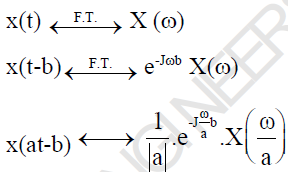QUESTION: 10

A pole zero pattern of a certain filter is shown in the figure. The filter must be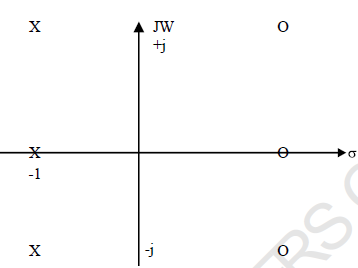Solution:

Since locations of poles and zeros are mirror image to each other about vertical axis, so it is an all pass filter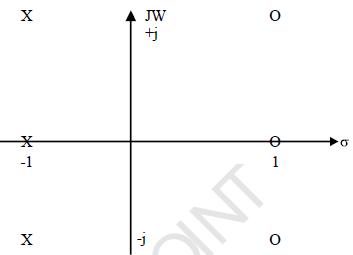QUESTION: 11

Determine the laplace-transform of signal x(t) shown in figure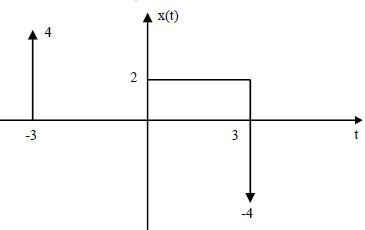Solution: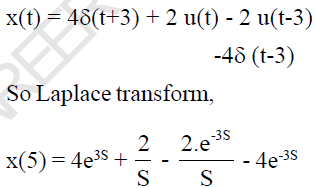QUESTION: 12

Determine the total energy of x(t) = 12 Sin (6t)

Solution: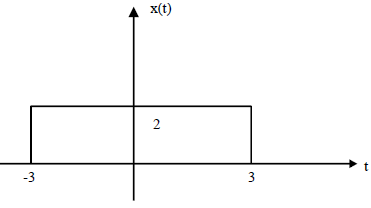x(t) = 12 Sinc (6t)
So, x(t) = 2 rect (f/6)
According to Parsvell's Theorem,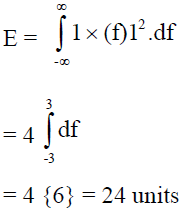QUESTION: 13

Determine the bandwidth of the signal x(t) = e-at u(t), so that it contains 90% of its total energy.

Solution: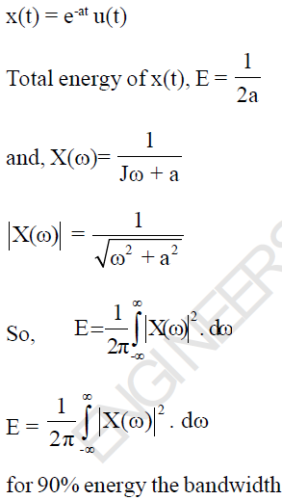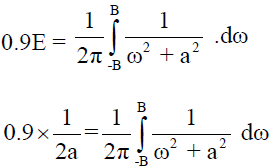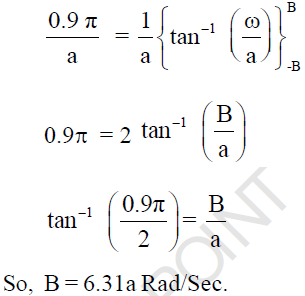QUESTION: 14

Determine the output y(t) for an input x(t) = e-2t u(t), if the step response of the system is t u(t).

Solution: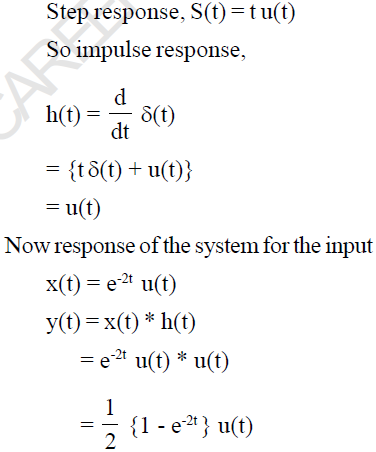QUESTION: 15

Simplify the following expression:
δ(-t) * u(t) * e-t/2 u(t)

Solution: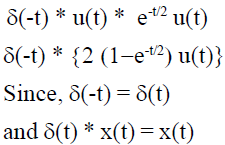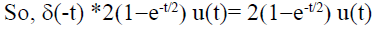QUESTION: 16

Consider the analog signal x(t) = 3 Cos 100πt. This signal is sampled with a frequency of 75 samples per second and at the reconstruction side an ideal LPF having cut-off frequency is 30Hz is used. Determine  the frequency component at the output of filter.

Solution: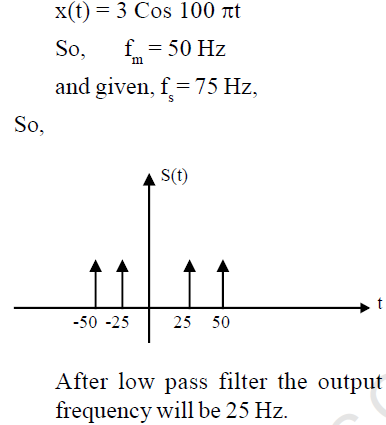QUESTION: 17

The input and output relationship of a system is given as,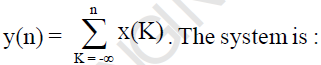Solution: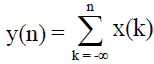It is the input-output relationship of an accumulator.
For any value of n, the output y(n) is depends only on the previous and present input
So it is causal.
It is also liner It is unstable system. Because the output is not bounded for the bounded input.

QUESTION: 18

A signal x(t) = 2 (1 - Cos2πt) is sampled with a sampling frequency of 10 Hz. Determine the z-transform of sampled signal.

Solution: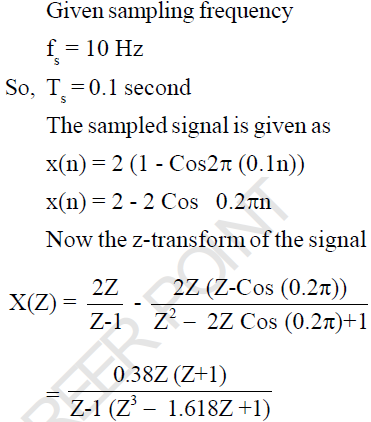QUESTION: 19

Given,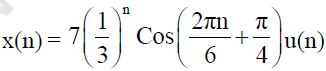Determine the ROC of its z-transform.

Solution: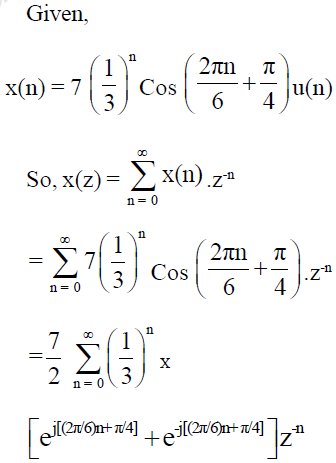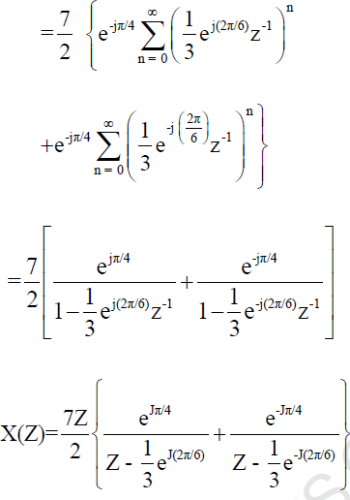ROC : |Z| > 1/3

QUESTION: 20

If a signal f(t) has energy E, the energy of the signal f(2t) is equal to

Solution: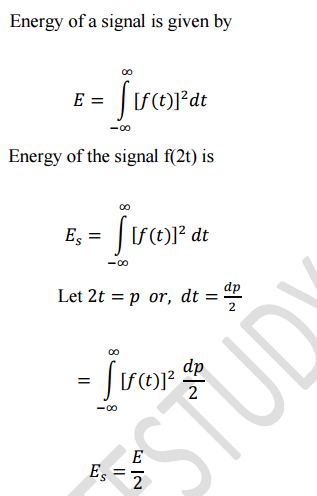QUESTION: 21

The correlation between two signals x1(t) and x2(t) is 6. If average power of x1(t) is 10 and x2(t) is 8.
Then determine the power of x1(t) + x2(t)

Solution:

Total energy is given as,
E = E1 + E2 + 2 Rx (τ)
= 10 + 8 + 2 × 6
= 30

QUESTION: 22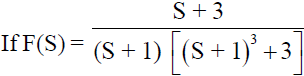, the co-efficient of term e-t in f(t) will be :

Solution: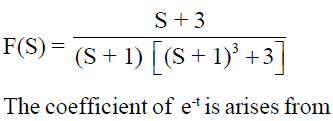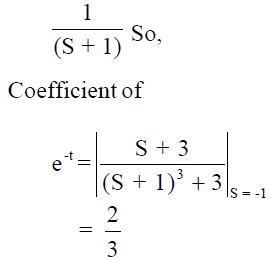QUESTION: 23

S1 : δ(n) is an energy signal having energy is 1.

S2 : u(n) is a power signal having power is 1/2.

Which of the above is/are correct?

Solution:

Energy of the signal is given as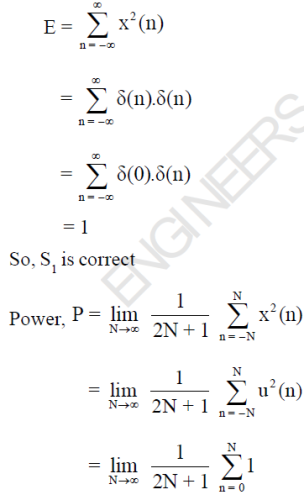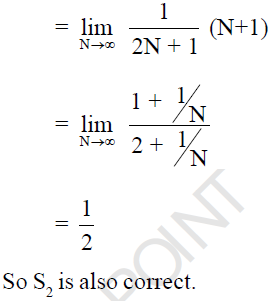QUESTION: 24

The input and output relationship of a system is given as,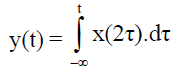The system is :

Solution: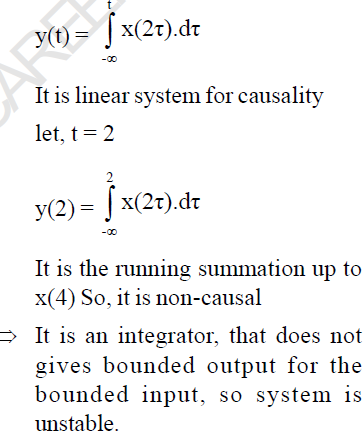QUESTION: 25

Determine the impulse response of the inverse system, if the impulse response of the system is u(n).

Solution: